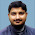## Sunday, October 19, 2008

### TRG Tech Mind Games

TRG Tech sometimes posts a "crack this code" challenge along with their job ads in local newspapers. This month the challenge is to tell what the following code does:

`z = lambda n:{ x for x in range (2, n+1) if len ([ i for i in range (2, x) if x%i == 0 ]) == 0 }`

I am not sure what functional language this is written in but it seems to be definition of a function which returns a set of prime numbers below the given number. However, I think there is a bug as well (not sure about the language, so I can't be 100% confident). The check in the list should be as following, in my opinion:

`[i for i in range (2, x-1) if x%i == 0]`

#### 2 comments:

1.This is a lambda function in Python language, which calculates prime numbers from 2 to n.

After declaring the above statement, you can call it by:
print z(6)

Its output will be null, because there is a logical error in the statement.

Range of x should be 2 to n and range of i should be 2 to x-1

Regards

2.This is a lambda function in Python which calculates prime numbers from 2 to n.

You can call it by:
print z(42)

Its output will be null because there is logical mistake in the statement. Range of i should be 2 to x-1 and more precisely range of x should be 2 to n.

Regards